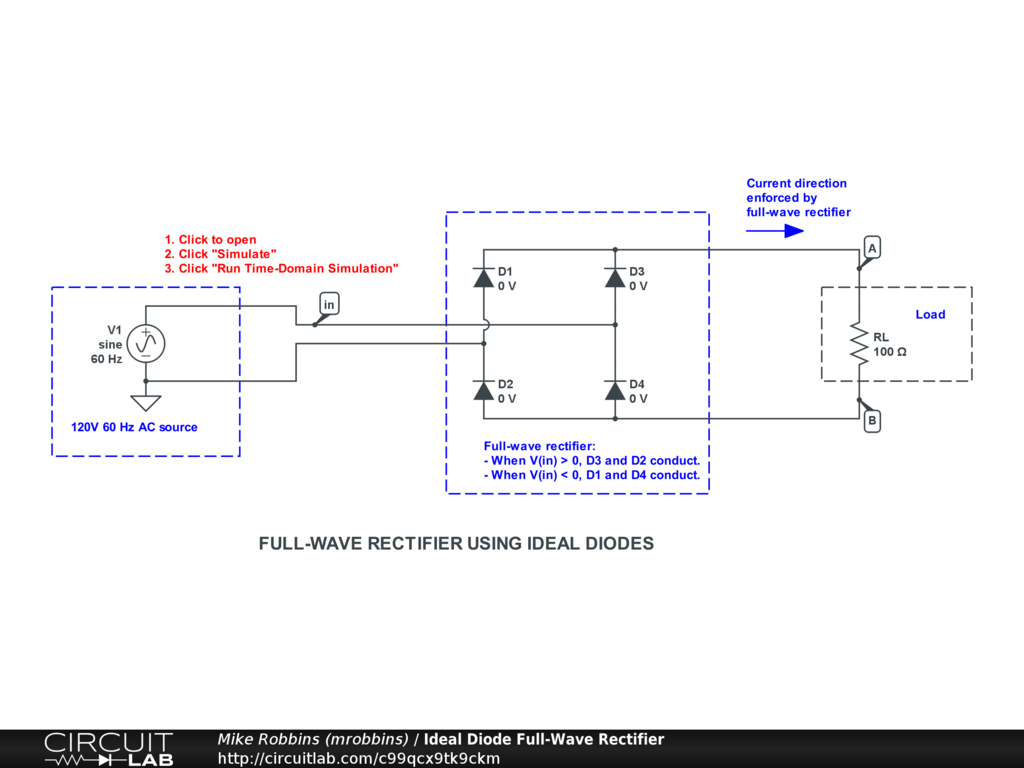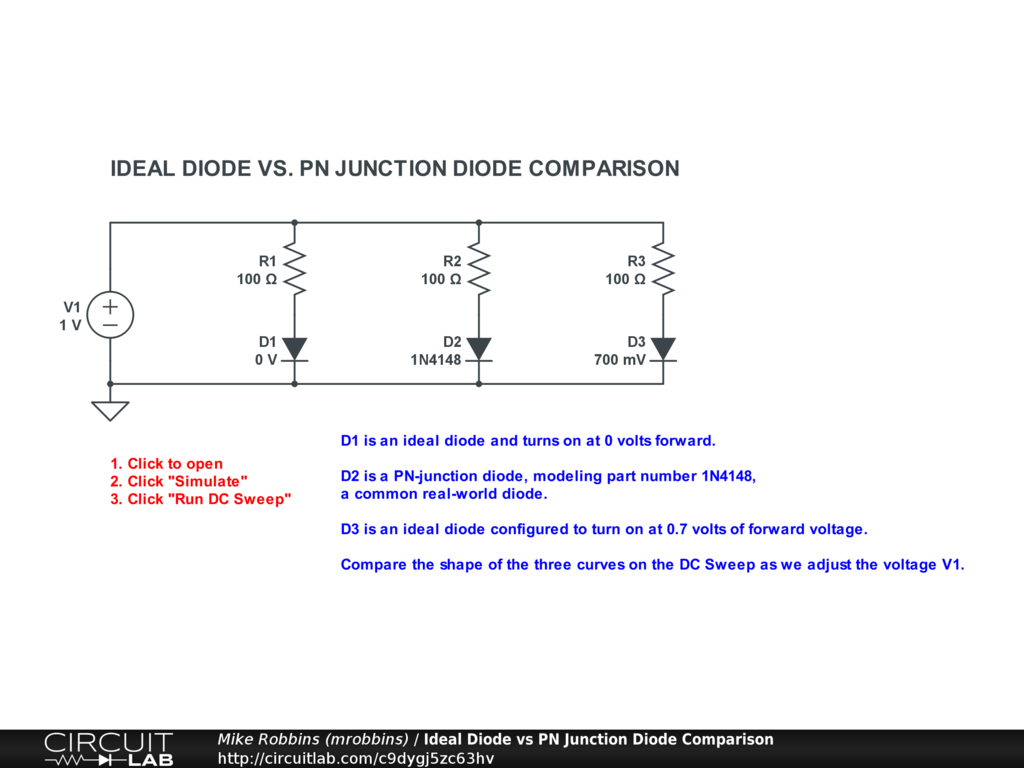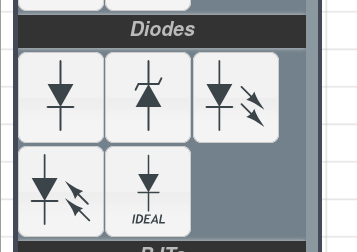## Ideal Diodes in CircuitLab

#### Aug 10 2020, 9:30 AM PDT · 0 comments »

We’re introducing a new component to the CircuitLab toolbox: the ideal diode.

We’ve had semiconductor PN junction diodes since we’ve launched, which show the exponential current-voltage relationship and accurately model real-world diodes.

In contrast, the ideal diode is more like a simulated on-off switch: the I-V curve would be piecewise linear. It acts like it’s open-circuit when reverse biased, and short-circuit when forward biased. You can simply drag the ideal diode from the toolbox into your circuit, and optionally double-click to configure its parameters.

Here’s an example using four ideal diodes to build a full-wave rectifier:Click to open and simulate the circuit above. Observe how the 4 diodes turn on and off at different times in the AC cycle.

Here’s an simulation comparing the regular PN Junction Diode with the ideal diode:Click to open and simulate the circuit above.

Note that D2 (a PN junction diode) gives us a gentle knee as it transitions from off to on, as real diodes do. D2’s curve is smooth in the calculus sense: its derivative is continuous.

In contrast, ideal diodes D1 and D3 show an abrupt (piecewise-linear) knee when they change from off to on. These are not smooth in the calculus sense: their derivatives are discontinuous.

### Use the PN junction diode when:

• Intending to accurately simulate real-world devices
• Simulation runtime is not a constraint

### Use the ideal diode when:

• Learning about signal rectification (where PN junction complexity is unnecessary)
• Simulating signal clamping behaviors (where hard clamping is acceptable)
• Simulating switching power supplies: buck, boost converters, etc. (where simulation performance is a constraint)
• Other cases where much faster-running simulations are preferred to accurate diode modeling

Both diode models, as well as Zener Diodes, photodiodes, and LEDs, are nows available in the CircuitLab toolbox: